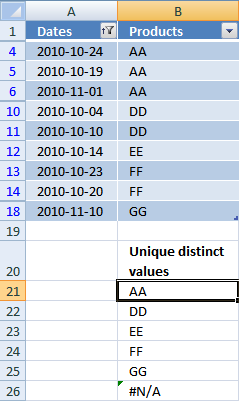Author: Oscar Cronquist Article last updated on January 31, 2019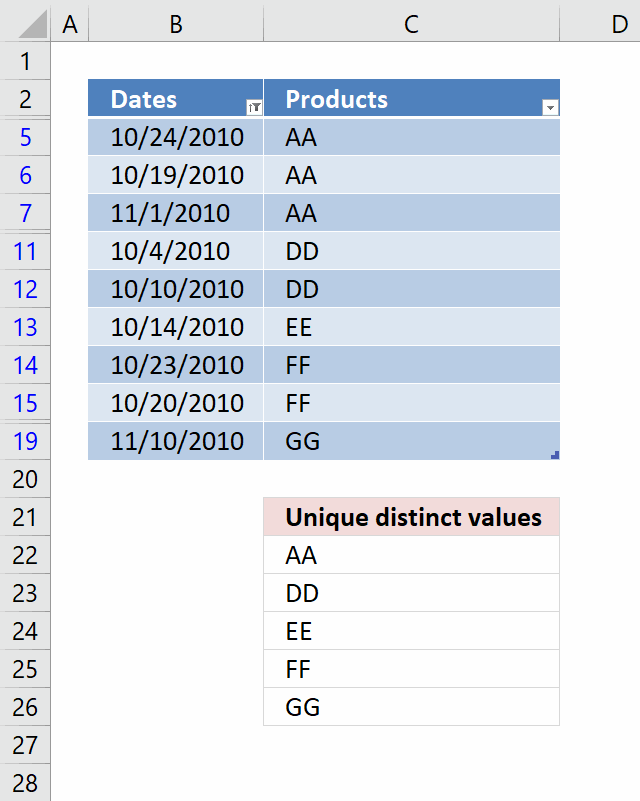This blog post demonstrates how to filter unique distinct values from an Excel table dynamically. When you change or add a filter to an excel table, the array formula extracts unique distinct values and displays them instantly.

Array formula in cell C22:

=INDEX(Table1[Products], MATCH(0, COUNTIF(\$C\$21:C21, Table1[Products])+(SUBTOTAL(3, OFFSET(Table1[Products], MATCH(ROW(Table1[Products]), ROW(Table1[Products]))-1, 0, 1))=0), 0))

#### How to create an array formula

1. Select a cell.
2. Copy (Ctrl + c) above array formula
3. Paste (Ctrl + v) array formula to selected cell.
4. Press and hold Ctrl + Shift simultaneously.
5. Press Enter once.
6. Release all keys.
Note, place your formula below or above the Excel defined Table. Rows are hidden when the Table is filtered and that could also hide parts of the array formula.

#### How to copy array formula

1. Copy cell C22 (CTRL + c).
2. Paste to cell range C23:C29 (CTRL + v).

### Explaining array formula in cell C22

The cell references to an Excel defined Table is different from regular cell references, Microsoft named them structured references and they look like this: Table1[Product]

They let you add or delete records to the Excel defined Table, however, the cell reference does NOT change so you don't need to adjust the references when your data set grows or shrinks.

#### Step 1 - Create an array of numbers

The ROW function returns the row number of a cell reference or an array of row numbers if the cell reference points to a cell range containing multiple cells.

The MATCH function changes the array of row numbers so it starts from 1 to n. n being the number of rows in the cell reference.

The array created in this step represents the row numbers of each item in the product column from 0 (zero) to n-1.

MATCH(ROW(Table1[Products]), ROW(Table1[Products]))-1

becomes

MATCH(ROW(C5:C19), ROW(C5:C19))-1

becomes

MATCH({5; 6; 7; 8; 9; 10; 11; 12; 13; 14; 15; 16; 17; 18; 19}, {5; 6; 7; 8; 9; 10; 11; 12; 13; 14; 15; 16; 17; 18; 19})-1

becomes

{1; 2; 3; 4; 5; 6; 7; 8; 9; 10; 11; 12; 13; 14; 15; 16; 17}-1

and returns

{0; 1; 2; 3; 4; 5; 6; 7; 8; 9; 10; 11; 12; 13; 14; 15; 16}

#### Step 2 - Create an array of arrays

This step is a workaround in order to be able to use the SUBTOTAL function with an array of values, the OFFSET function allows you to create an array of arrays.

Note, the OFFSET function is volatile meaning it can slow down your workbook if used extensively.

Each array contains a single value, this will allow the SUBTOTAL function handle the array as a whole and process all values.

OFFSET(Table1[Products], MATCH(ROW(Table1[Products]), ROW(Table1[Products]))-1, 0, 1)

becomes

OFFSET(Table1[Products], {0; 1; 2; 3; 4; 5; 6; 7; 8; 9; 10; 11; 12; 13; 14; 15; 16}, 0, 1)

and returns

{"AA";"AA";"AA";"AA";"BB";"CC";"DD";"DD";"EE";"FF";"FF";"FF";"GG";"GG";"GG"}

#### Step 3 - Identify hidden values

The first argument in the SUBTOTAL function is 3 representing the COUNTA function. It counts cells that are not empty. A 0 (zero) means that the value is hidden and 1 is visible.

SUBTOTAL(3, OFFSET(Table1[Products], MATCH(ROW(Table1[Products]), ROW(Table1[Products]))-1, 0, 1))=0

becomes

SUBTOTAL(3, {"AA";"AA";"AA";"AA";"BB";"CC";"DD";"DD";"EE";"FF";"FF";"FF";"GG";"GG";"GG"})=0

becomes

{0; 0; 1; 1; 1; 0; 0; 0; 1; 1; 1; 1; 1; 0; 0; 0; 1}=0

and returns

{TRUE; TRUE; FALSE; FALSE; FALSE; TRUE; TRUE; TRUE; FALSE; FALSE; FALSE; FALSE; FALSE; TRUE; TRUE; TRUE; FALSE}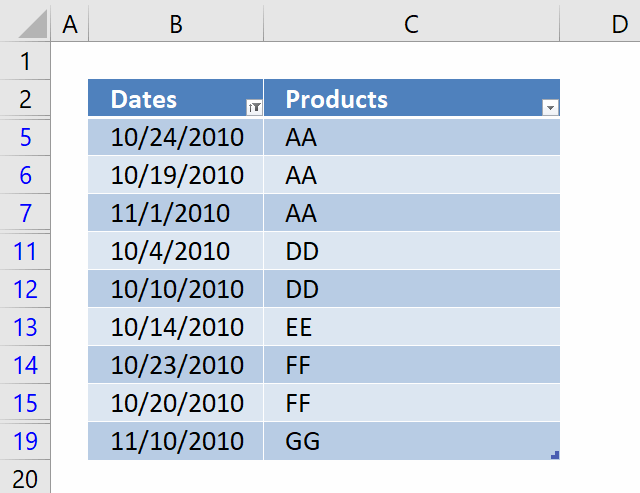The first value in the array is TRUE meaning it is hidden, the first row in the Excel defined Table is 3 which is hidden etc.

#### Step 4 - Identify values shown in cells above current cell

The COUNTIF function counts values based on a condition or criteria, the first argument contains an expanding cell reference, it grows when the cell is copied to cells below. This makes the formula aware of values displayed in cells above. 0 (zero) indicates values that not yet have been displayed.

COUNTIF(\$C\$21:C21,Table1[Products])

becomes

COUNTIF(\$C\$21:C21,C5:C19)

and returns

{0; 0; 0; 0; 0; 0; 0; 0; 0; 0; 0; 0; 0; 0; 0; 0; 0}.

#### Step 5 - Add arrays

This step applies OR logic to the formula meaning if a value has not yet been displayed OR the value is filtered (not hidden) in the Excel defined Table.

COUNTIF(\$C\$21:C21, Table1[Products])+(SUBTOTAL(3, OFFSET(Table1[Products], MATCH(ROW(Table1[Products]), ROW(Table1[Products]))-1, 0, 1))=0)

becomes

{0; 0; 0; 0; 0; 0; 0; 0; 0; 0; 0; 0; 0; 0; 0; 0; 0}+(SUBTOTAL(3, OFFSET(Table1[Products], MATCH(ROW(Table1[Products]), ROW(Table1[Products]))-1, 0, 1))=0)

becomes

{0; 0; 0; 0; 0; 0; 0; 0; 0; 0; 0; 0; 0; 0; 0; 0; 0}+{TRUE; TRUE; FALSE; FALSE; FALSE; TRUE; TRUE; TRUE; FALSE; FALSE; FALSE; FALSE; FALSE; TRUE; TRUE; TRUE; FALSE}

and returns

{1; 1; 0; 0; 0; 1; 1; 1; 0; 0; 0; 0; 0; 1; 1; 1; 0}.

#### Step 6 - Find the position of a value meeting both conditions

The MATCH function returns the position in a cell range or array based on a given value.

MATCH(lookup_value, lookup_array, [match_type])

MATCH(0, COUNTIF(\$C\$21:C21, Table1[Products])+(SUBTOTAL(3, OFFSET(Table1[Products], MATCH(ROW(Table1[Products]), ROW(Table1[Products]))-1, 0, 1))=0), 0)

becomes

MATCH(0, {1; 1; 0; 0; 0; 1; 1; 1; 0; 0; 0; 0; 0; 1; 1; 1; 0}, 0)

and returns 3. If there are multiple values matching then return the position of the first instance.

Note, remember to use 0 (zero) in the third argument [match_type] in the MATCH function in order to perform an exact match.

#### Step 7 - Return value

The INDEX function returns a value based on a cell reference and column/row numbers.

INDEX(Table1[Products], MATCH(0, COUNTIF(\$C\$21:C21, Table1[Products])+(SUBTOTAL(3, OFFSET(Table1[Products], MATCH(ROW(Table1[Products]), ROW(Table1[Products]))-1, 0, 1))=0), 0))

becomes

INDEX(Table1[Products], 3)

and returns "AA" in cell C22.

#### How to create an Excel Defined Table

1. Select a cell in your data set.
2. Press short cut keys CTRL + T to convert the data set to an Excel defined Table.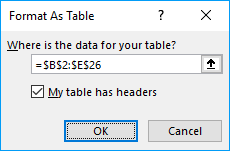3. Click checkbox "My table has headers" if they exist.
4. Click OK button.

#### How to apply a filter to the Excel Defined Table

Click on black arrow near header "Dates".
Deselect year 2011.
Click Ok.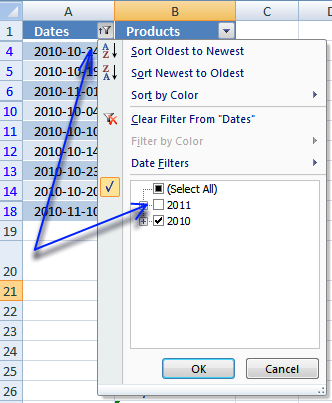The array formula is instantly refreshed.